## Temperature Effect on Semiconductor Diode:

Diode Power Dissipation – The power dissipation in a diode is simply calculated as the device terminal voltage multiplied by the current level.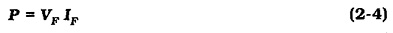Device manufacturers specify a maximum power dissipation for each type of diode. If the specified level is exceeded, the device will overheat and it may short-circuit or open-circuit.

The maximum power that may be dissipated in a diode (or any other electronic device) is normally specified for an ambient temperature of 25°C, or sometimes for a 25°C case temperature. When the Temperature Effect on Semiconductor Diode exceeds the specified level, the device maximum power dissipation must be derated.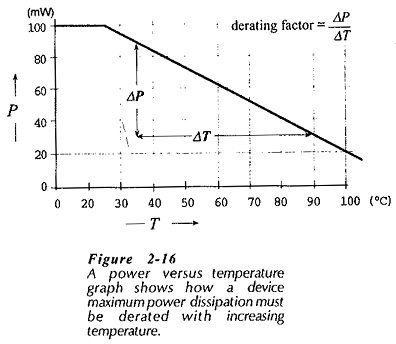Figure 2-16 shows the type of power-versus-temperature graph provided on some device data sheets. The maximum power dissipation for any given Temperature Effect on Semiconductor Diode can simply be read from the graph. Then, the maximum level of forward current is calculated from Eq. 2-4. Instead of a power-versus-temperature graph, the rectifier diode data has a current-versus-­temperature graph. This allows the maximum forward current at a desired temperature to be determined directly.

As an alternative to a power or current graph, a derating factor is sometimes listed on a device data sheet. As illustrated in Fig. 2-16, the derating factor defines the slope of the power-versus­-temperature graph. It can be used to draw the graph, or employed directly without reference to the graph. The equation for the new maximum power dissipation when the temperature changes involves the specified power at the specified temperature (P1 at T1), the temperature change (ΔT), and the derating factor.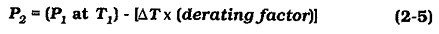### Forward Voltage Drop

There are circumstances in which it is important to know the precise level of a diode forward voltage drop. In these cases, the graphical analysis techniques discussed already may be appropriate. However, in Fig. 2-17, the voltage drop across a forward-biased pn-junction changes with Temperature Effect on Semiconductor Diode by approximately -1.8 mV/°C for a silicon device, and by -2.02 mV/°C for germanium. A diode VF at any temperature can be calculated from a knowledge of VF at the starting temperature (VF1 at T1), the temperature change (ΔT), and the voltage/temperature coefficient (ΔVF/°C).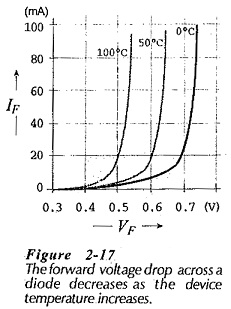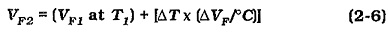### Dynamic Resistance:

Equation 2-2 for calculating the dynamic resistance of a forward biased diode is correct only for 25°C junction temperatures. For higher or lower temperatures, the equation must be modified to,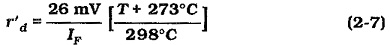where T is the junction temperature in degrees Celsius.

Scroll to Top